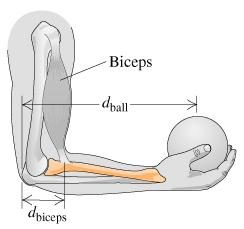# Problem: When you lift an object by moving only your forearm, the main lifting muscle in your arm is the biceps. Suppose the mass of a forearm is 1.00 kg. If the biceps is connected to the forearm a distance dbiceps = 3.50 cm from the elbow, the force Fbiceps = 113.96 N is exerted to hold a 600 g ball at the end of the forearm at distance dball = 37.0 cm from the elbow, with the forearm parallel to the floor.Which of the following should the forearm be modeled as?A. a particle moving with constant accelerationB. a flexible rod moving with constant accelerationC. a rigid rod moving with constant accelerationD. a particle in equilibriumD.a flexible rod in equilibriumE.a rigid rod in equilibrium

###### FREE Expert Solution

The forearm is in equilibrium. Therefore, the acceleration is zero.

91% (259 ratings)###### Problem Details

When you lift an object by moving only your forearm, the main lifting muscle in your arm is the biceps. Suppose the mass of a forearm is 1.00 kg. If the biceps is connected to the forearm a distance dbiceps = 3.50 cm from the elbow, the force Fbiceps = 113.96 N is exerted to hold a 600 g ball at the end of the forearm at distance dball = 37.0 cm from the elbow, with the forearm parallel to the floor.Which of the following should the forearm be modeled as?

A. a particle moving with constant acceleration

B. a flexible rod moving with constant acceleration

C. a rigid rod moving with constant acceleration

D. a particle in equilibrium

D.a flexible rod in equilibrium

E.a rigid rod in equilibrium